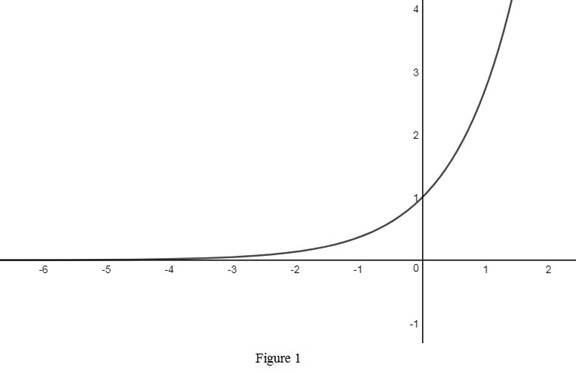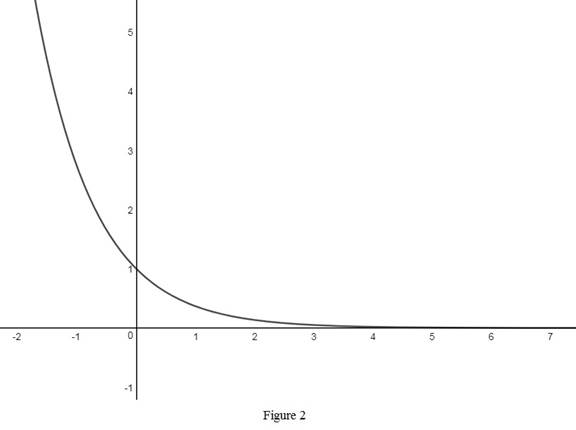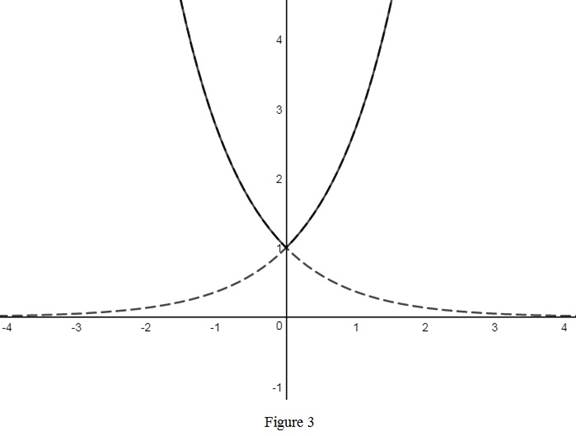# The rough graph of the function y = e | x | use transformations if needed.### Single Variable Calculus: Concepts...

4th Edition
James Stewart
Publisher: Cengage Learning
ISBN: 9781337687805### Single Variable Calculus: Concepts...

4th Edition
James Stewart
Publisher: Cengage Learning
ISBN: 9781337687805

#### Solutions

Chapter 1.5, Problem 14E
To determine

## To sketch: The rough graph of the function y=e|x| use transformations if needed.

Expert Solution

### Explanation of Solution

The standard graph of the function y=ex is roughly sketched as shown below in Figure 1.Reflect the graph y=ex about y-axis and obtain the graph of y=ex . Thus, the graph of y=ex is drawn and shown below in Figure 2.Then, to draw the graph of y=e|x| , remove the portions of the graphs that appear below 1. Thus, the graph of y=e|x| is shown below in Figure 3.Observe that Figure 3 is obtained from Figure 1 and Figure 2 in such a way that the portions that appear below 1 are removed.

### Have a homework question?

Subscribe to bartleby learn! Ask subject matter experts 30 homework questions each month. Plus, you’ll have access to millions of step-by-step textbook answers!